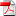# Deep neural networks algorithms for stochastic control problems on finite horizon, Part 2: numerical applications - A. Bachouch, C. Huré, N. Langrené, H. Pham

18
Jan

This paper presents several numerical applications of deep learning-based algorithms that have been analyzed in . Numerical and comparative tests using TensorFlow illustrate the performance of our different algorithms, namely control learning by performance iteration (algorithms NNcontPI and ClassifPI), control learning by hybrid iteration (algorithms Hybrid-Now and Hybrid-LaterQ), on the 100-dimensional nonlinear PDEs examples from  and on quadratic Backward Stochastic Differential equations as in . We also provide numerical results for an option hedging problem in finance, and energy storage problems arising in the valuation of gas storage and in microgrid management.

•RR-FIME-18-11